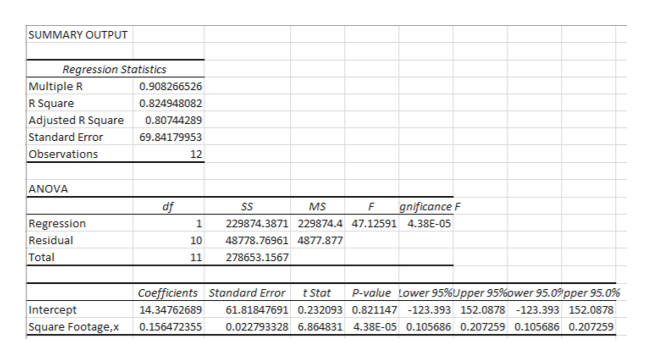Question

in the attached, I only need help with the last three parts. E, G, and H

thanks

check_circleExpert Solution
Step 1

According to the provided data, the square footage is the explanatory variable and selling price is the dependent variable.

(e) The least square regression line is obtained using Excel by following the below steps:

1. Enter the data in the spreadsheet.
2. Go to Data>Data Analysis>select “Regression”.
3. Enter the required values in the columns.
4. Click OK to obtain the result.help_outlineImage TranscriptioncloseSUMMARY OUTPUT Regression Statis tics Multiple R R Square 0.908266526 0.824948082 Adjusted R Square Standard Error Observations 0.80744289 69.84179953 12 ANOVA df SS MS gnificance F F Regression Residual 1 229874.3871 229874.4 47.12591 4.38E-05 10 48778.76961 4877.877 Total 11 278653.1567 Lower 95%Upper 95 % ower 95.0pper 95.0% Coefficients Standard Error P-value t Stat Intercept Square Footage,x 14.34762689 61.81847691 0.232093 0.821147 -123.393 152.0878 -123.393 152.0878 0.156472355 0.022793328 6.864831 4.38E-05 0.105686 0.207259 0.105686 0.207259 fullscreen
Step 2

From the obtained output, the least square regression line is,

Step 3

(g) The y intercept can be defined as the expected mean value of y when the value of x is equal to 0.

It is not making any sense having the selling pri...

### Want to see the full answer?

See Solution

#### Want to see this answer and more?

Solutions are written by subject experts who are available 24/7. Questions are typically answered within 1 hour*

See Solution
*Response times may vary by subject and question
Tagged in

### Other